xFundamental frequencyEncyclopediaThe fundamental frequency, often referred to simply as the fundamental and abbreviated f0, is defined as the lowest frequency of a periodic waveform
Waveform
Waveform means the shape and form of a signal such as a wave moving in a physical medium or an abstract representation.In many cases the medium in which the wave is being propagated does not permit a direct visual image of the form. In these cases, the term 'waveform' refers to the shape of a graph...

. In terms of a superposition of sinusoids (e.g. Fourier series
Fourier series
In mathematics, a Fourier series decomposes periodic functions or periodic signals into the sum of a set of simple oscillating functions, namely sines and cosines...

), the fundamental frequency is the lowest frequency sinusoidal in the sum.

All sinusoidal and many non-sinusoidal waveforms are periodic, which is to say they repeat exactly over time. A single period is thus the smallest repeating unit of a signal, and one period describes the signal completely. We can show a waveform is periodic by finding some period T for which the following equation is true: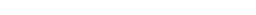Where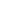is the function of the waveform.

This means that for multiples of some period T the value of the signal is always the same. The lowest value of T for which this is true is called the fundamental period (T1) and thus the fundamental frequency (F0) is given by the following equation:Where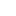is the fundamental frequency and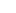is the fundamental period.

The fundamental frequency of a sound wave in a tube with a single CLOSED end can be found using the following equation: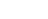L can be found using the following equation: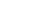λ (lambda
Lambda
Lambda is the 11th letter of the Greek alphabet. In the system of Greek numerals lambda has a value of 30. Lambda is related to the Phoenician letter Lamed . Letters in other alphabets that stemmed from lambda include the Roman L and the Cyrillic letter El...

) can be found using the following equation: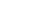The fundamental frequency of a sound wave in a tube with either both ends OPEN or both ends CLOSED can be found using the following equation: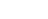L can be found using the following equation: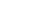The wavelength, which is the distance in the medium between the beginning and end of a cycle, is found using the following equation:Where:= fundamental frequency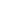= length of the tube= velocity of the sound wave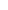= wavelength

At 20 °C (68 °F) the speed of sound
Speed of sound
The speed of sound is the distance travelled during a unit of time by a sound wave propagating through an elastic medium. In dry air at , the speed of sound is . This is , or about one kilometer in three seconds or approximately one mile in five seconds....

in air is 343 m/s (1129 ft/s). This speed is temperature dependent and does increase at a rate of 0.6 m/s for each degree Celsius increase in temperature (1.1 ft/s for every increase of 1 °F).

The velocity of a sound wave at different temperatures:-
• v = 343.2 m/s at 20 °C
• v = 331.3 m/s at 0 °C

## Mechanical systems

Consider a beam, fixed at one end and having a mass attached to the other; this would be a single degree of freedom (SDoF) oscillator. Once set into motion it will oscillate at its natural frequency. For a single degree of freedom oscillator, a system in which the motion can be described by a single coordinate, the natural frequency depends on two system properties: mass and stiffness. The radian frequency, ωn, can be found using the following equation: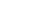Where:

k = stiffness
Stiffness
Stiffness is the resistance of an elastic body to deformation by an applied force along a given degree of freedom when a set of loading points and boundary conditions are prescribed on the elastic body.-Calculations:...

of the beam

m = mass of weight

From the radian frequency, the natural frequency, fn, can be found by simply dividing ωn by 2π. Without first finding the radian frequency, the natural frequency can be found directly using: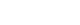Where:

fn = natural frequency in hertz (cycles/second)

k = stiffness of the beam (Newtons/Meter or N/m)

m = mass at the end (kg)

while doing the modal analysis of structures and mechanical equipments, the frequency of 1st mode is called fundamental frequency.

• Missing fundamental
Missing fundamental
A sound is said to have a missing fundamental, suppressed fundamental, or phantom fundamental when its overtones suggest a fundamental frequency but the sound lacks a component at the fundamental frequency itself....

• Natural frequency
• Oscillation
Oscillation
Oscillation is the repetitive variation, typically in time, of some measure about a central value or between two or more different states. Familiar examples include a swinging pendulum and AC power. The term vibration is sometimes used more narrowly to mean a mechanical oscillation but sometimes...

• Hertz
Hertz
The hertz is the SI unit of frequency defined as the number of cycles per second of a periodic phenomenon. One of its most common uses is the description of the sine wave, particularly those used in radio and audio applications....

• Electronic tuner
Electronic tuner
The term electronic tuner can refer to a number of different things, depending which discipline you wish to study.In the Discipline of radio frequency electronics an electronic tuner is a device which tunes across a part of the radio frequency spectrum by the application of a voltage or appropriate...

• Scale of harmonics
Scale of harmonics
The scale of harmonics is a musical scale based on the noded positions of the natural harmonics existing on a string. This musical scale is present on the guqin, regarded as one of the first string instruments with a musical scale . Most fret positions appearing on Non-Western string instruments ...

• Pitch detection algorithm
Pitch detection algorithm
A pitch detection algorithm is an algorithm designed to estimate the pitch or fundamental frequency of a quasiperiodic or virtually periodic signal, usually a digital recording of speech or a musical note or tone. This can be done in the time domain or the frequency domain.PDAs are used in various...